Home
Then and Now:  A-B
Then and Now:  B-C
Then and Now:  C
Then and Now:  D-E
Then and Now:  F-G
Then and Now:  G
Then and Now:  H
Then and Now:  H
Then and Now:  J-K
Then and Now:  L
Then and Now:  M
Then and Now:  M
Then and Now:  N-O
Then and Now:  P
Then and Now:  R
Then and Now:  S
Then and Now:  T
Then and Now:  V-Z

### Then and Now:  J-K

Pictures from our What's What; pictures of us today.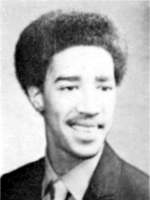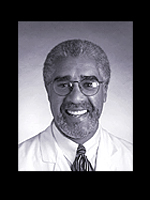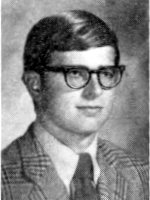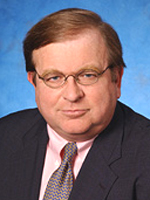Dave Jacobs Chip Juan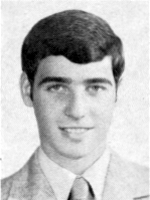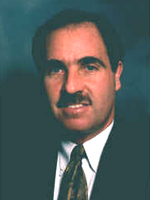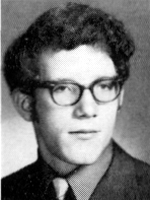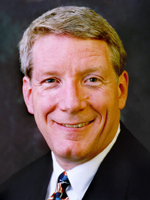Sumner Karas Steve Kelley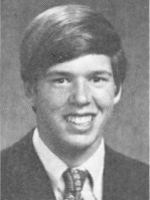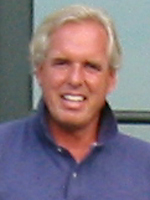Peter Kiernan